# Formulae for GeneratingAll Prime Numbers

by Charles Dean Pruitt
(with trigonometric prompting by Katya A.)
ABSTRACT:
The following is a method to produce equations of various complexities
with real coeffecients whose solutions generate all prime numbers in an interval.

METHOD:
From theory and by inspection, composite numbers with similar
factors appear on the number line in a periodic fashion.
Conversely prime numbers appear on the number line irregularly as "not composites".
Therefore it is possible to find a periodic function such as a sine curve or cycloid with
a particular periodicity which will cross the x-axis (on a two-dimensional Cartesian
coordinate system) at each integer whose factors correspond to that periodicity.
For purposes of this argument the sine curve is used because it is the most familiar.
For example: the sine curve (Sin xPi/2) has an amplitude of one and passes through
the points on the x-axis (0,0), (2,0), (4,0), (6,0) to infinity, i.e. multiples of two.Figure 1.Equation A. y = (Sin xPi/2)

Likewise the sine curve (Sin xPi/3) has an amplitude of one and passes through
the points on the x-axis (0,0), (3,0), (6,0), (9,0) to infinity, i.e. multiples of three.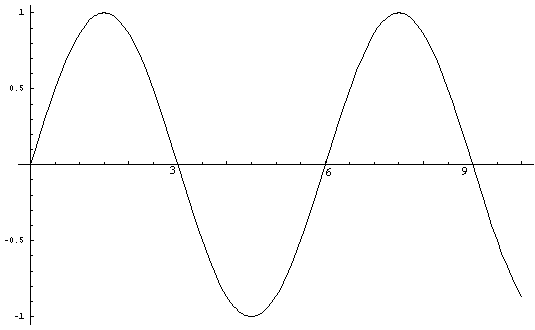Figure 2. Equation B. y = (Sin xPi/3)

In the same way, the sine curve (Sin xPi/5) has an amplitude of one and passes through
the points on the x-axis (0,0), (5,0), (10,0), (15,0) to infinity, i.e. multiples of five.Figure 3. Equation C. y = (Sin xPi/5)

From each of the above three figures it can be seen that the sine curve passes through
all points on the x-axis which have its respective prime (and periodicity) as a factor.
When Figure 1 is multiplied by Figure 2, Figure 4 results.
This is the graph of the curve (Sin xPi/2 * Sin xPi/3).
It is possible to see that the zero points where the curve crosses the x-axis
correspond to the integers which have 2 or 3 or both as a factor.
Where the curve does not intersect the x-axis at the integer values
are integers relatively prime to two or three.
This is the case up until 'x' equals 25 or five squared.
This is consistent with standard number theory concerning factorability.
Therefore for the equation
Sin xPi/2 * Sin xPi/3 = 0,
the points which satisfy the equation for 'x' are
(2,0), (3,0), (4,0), (6,0), (8,0), (9,0), (10,0), (12,0), (14,0), (15,0),
(16,0), (18,0), (20,0), (21,0), (22,0), (24,0).
By inspection (see Figure 4), it can be seen that there is no intersection with
the curve at the points on the x-axis
(5,0), (7,0), (11,0), (13,0), (17,0), (19,0), (23,0).
This is confirmed when these values for 'x' are substituted into
the equation and non-zero answers result.
In effect these are all the primes less than five squared except two and three.
By the above method is it possible to generate equations which when solved for
x =0 indicate by elimination that all integers left over are primes.Figure 4. Equation D. y = (Sin xPi/2 * Sin xPi/3)

In order to find the equation which will provide the curve which
will cross the x-axis for all non-primes less than seven squared,
it is necessary to multiply (Sin xPi/2 * Sin xPi/3 * Sin xPi/5).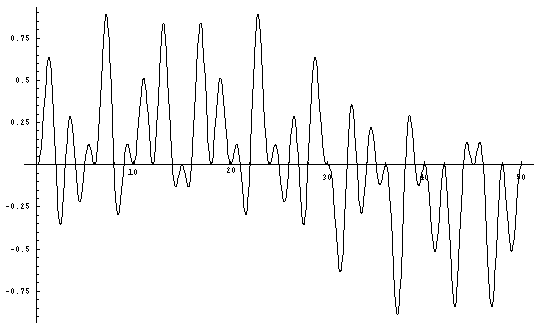Figure 5. equation E.y = (Sin xPi/2 * Sin xPi/3 * Sin xPi/5)

Although it is not as clear as on the other graphs,
it is possible to recognize the prime points on the x-axis.
Therefore when solving for
Sin xPi/2 * Sin xPi/3 * Sin xPi/5 = 0,
the solutions for 'x' (when x<49) comprise all the composite numbers
less than 49 (7 squared) as well as 2, 3, and 5.
Therefore all the remaining integers are prime.
With 2, 3, and 5 this constitutes all the primes less than 49.
It is possible to check this empirically by substitution.
For exceedingly large samples it is not useful to view a whole graph
because the fine details are not readily clear.
However it is possible to calculate for a large range
by multiplying all the consecutive sine curves together.
In the following example the first 27 sine curves (Sin xPi/2) through (Sin xPi/103)
were multiplied together to yield a graph for the resultant curve
of which 100 units are shown below (figure 6).
That curve is stated as:
y = [Sin xPi/2 * Sin xPi/3 * Sin xPi/5 * Sin xPi/7 * Sin xPi/11 * Sin xPi/13 * Sin xPi/17 *
Sin xPi/19 * Sin xPi/23 * Sin xPi/29 * Sin xPi/31 * Sin xPi/37 * Sin xPi/41 * Sin xPi/43 *
Sin xPi/47 * Sin xPi/53 * Sin xPi/59 * Sin xPi/61 * Sin xPi/67 * Sin xPi/71 * Sin xPi/73 *
Sin xPi/79 * Sin xPi/83 * Sin xPi/89 *Sin xPi/97 *Sin xPi/101 * Sin xPi/103]
(Equation F.)
Solving the resultant equation for zero yields all the
composite numbers up to 103 squared (10609) and
consequently by elimination all of the primes less than 10609.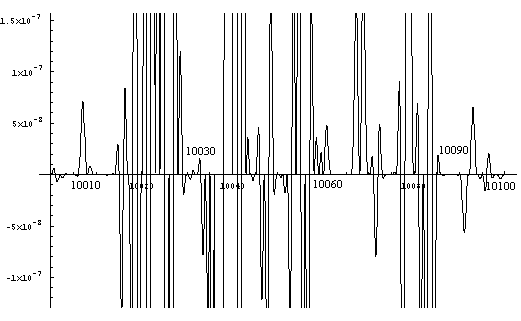Figure 6. Equation F. y = (Sin xPi/2* Sin xPi/3 * ... * Sin xPi/101 * Sin xPi/103)

For purposes of illustration a small segment of the graph
is blown up (figure 7) to shown the fine detail and confirm
visually that indeed the resulting curve crosses the x-axis
at all the composite numbers and not at any of the primes.
However for all values of 'x' which satisfy the equation,
results can only be derived by solving for zero.Figure 7. Equation F. Detail 10000 to 10010

It appears from this segment of the graph that 10007 and 10009
are prime (and constitute a prime pair). This is indeed the case.
The curve between 10004 and 10006 has an amplitude of less than one ten-billionth.
With further magnification (see Figure 8) details as fine one part in a trillion
are resolved and indicate 10005 is composite.
(Of course there are easier methods to determine
compositeness of numbers ending in 5.)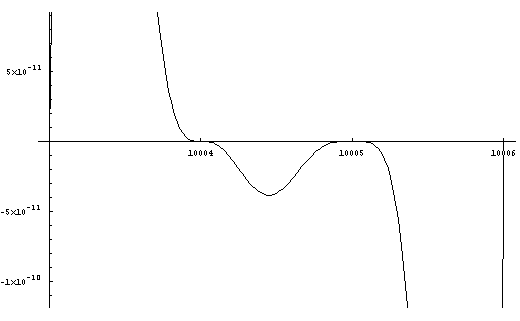Figure 8. Equation F. Detail 10003-10006

PROOF

Each periodic function as defined above intersects
the x-axis at points that correspond to all
the integers divisible by that function when y=0.
Sin xPi/2=0 represents all integers divisible by 2.
Sin xPi3=0 represents all integers divisible by 3.
Sin xPi/5=0 represents all integers divisible by 5.
When two functions are multiplied together,
they will show up all the zeroes because 0 times anything is 0 .
Sin xPi/2 * Sin xPi/3=0 represents all integers divisible by 2 and/or 3.
Sin xPi/3 * Sin xPi/5=0 represents all integers divisible by 3 and/or 5.
Sin xPi/5 * Sin xPi/7=0 represents all integers divisible by 5 and/or 7.
There is not duplication because 0 times 0 is 0
and hence there would be only one point indicated instead of two.
Likewise the same argument applies when many sine curves are multiplied together.
When all sine curves (each with periodicity corresponding to a prime and
all such curves up to and including that whose periodicity is
less than or equal to the square root of the largest number
in the sample space being tested) are multiplied together,
the resultant equation gives each composite number one time
when the equation is solved for zero.
Discarding these from the set of integers leaves the primes.
In practice this has become a formulaic Sieve of Erastathenes.
This not a true equation whose solutions yield primes.
To do this it is necessary to choose periodic equations
which are not as tidy as a Sine curve.
This is done by using a similar curve (in this case a Cosine curve)
and translating the axes. According to the parameters set out above,
a necessary condition is that the curve intersects the x-axis in a periodic fashion
and at the points where it intersects exhibits a desired characteristic.
In this case relative primality.
Consider the case of relative primality with respect to two.
This is straightforward since it is possible to construct a curve that intersects
the x-axis at points relatively prime wih respect to two (i.e. 1, 3, 5, 7, etc.).
Below is a Cosine curve with the axes translated such that the troughs of the
Cosine curve intersect the x-axis at integers relatively prime to two.
The equation of this curve is y = 1+Cos(Pi(x))Figure 9. Equation G. y = 1+Cos(Pi(x))

From Figure 9 it is possible to see that for x = 0, y assumes values relatively prime to 2.
Likewise it is possible to choose similar Cosine curves for other integers.
However to do this for three for example, it is necessary to use multiple curves since a
single Cosine curve will not touch the x-axis at every integer relatively prime to three.
The first curve is y = 1+Cos(Pi(2x+5)/3)Figure 10. Equation H. y = 1+Cos(Pi(2x+5)/3)

Observe that this curve intersects the x-axis at values 3a + 2
and are all relatively prime to 3.
The second curve is y = 1+Cos(Pi(2x+7)/3)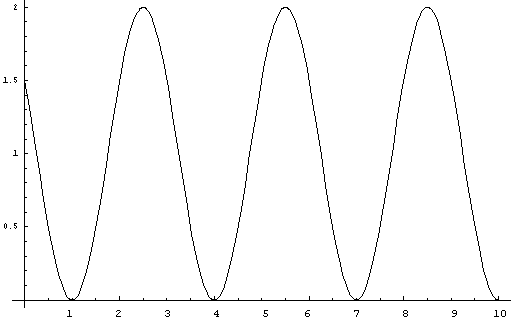Figure 11. Equation I. y = 1+Cos(Pi(2x+7)/3)

Observe that this curve intersects the x-axis at values 3a + 1
and are all relatively prime to 3.
When y = 1+Cos(Pi(2x+5)/3) and y = 1+Cos(Pi(2x+7)/3)
are multiplied together the equation is
y = 1 + Cos(Pi2x+5)/3) + Cos(Pi(2x+7)/3) + Cos(Pi(2x+5)/3)*Cos(Pi(2x+7)/3).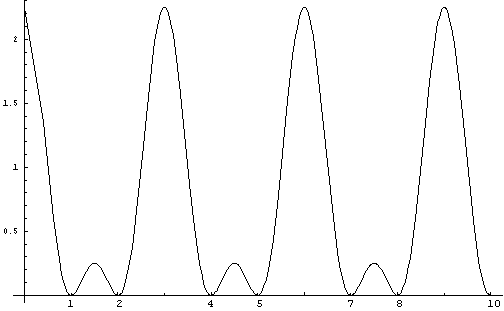Figure 12. Equation J.
y = 1 + Cos(Pi(2x+5)/3) + Cos(Pi(2x+7)/3) + Cos(Pi(2x+5)/3)*Cos(Pi(2x+7)/3)

Observe that this curve intersects the x-axis at values 3a + 1 and 3a + 2
and are all relatively prime to 3.
To get an equation which when solved for y = 0 yields values of "x" which are
relatively prime to two and three it is necessary to add Equation G and Equation J.
If y = 1 + Cos(Pi(2x+5)/3) + Cos(Pi(2x+7)/3) + Cos(Pi(2x+5)/3)*Cos(Pi(2x+7)/3)
is added to y = 1+Cos(Pi(x) the resulting equation is
y = 2+ Cos(Pi(2x+5)/3) + Cos(Pi(2x+7)/3) + Cos(Pi(x)) + Cos(Pi(2x+5)/3)*Cos(Pi(2x+7)/3).
When solved for y = 0, this equation gives values relatively prime to infinity and
gives prime values for "x" when y = 0 when x<5².
As is readily apparent, this equation is unwieldy and
not amenable for searching for primes.
The graph of this equation is: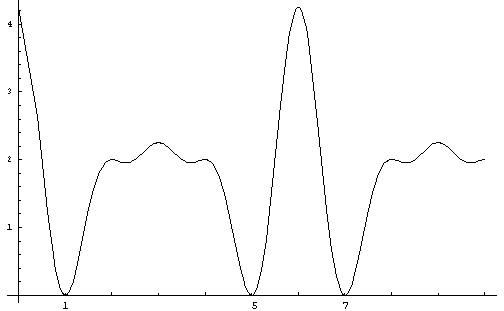Figure 13. Equation K.
y = 2+ Cos(Pi(2x+5)/3) + Cos(Pi(2x+7)/3) + Cos(Pi(x)) + Cos(Pi(2x+5)/3)*Cos(Pi(2x+7)/3)

The corresponding equations which describe a curve on which the zero points with
respect to "y" are relatively prime to five are derived from the following equations:
y = 1+Cos(Pi(2x+7)/5)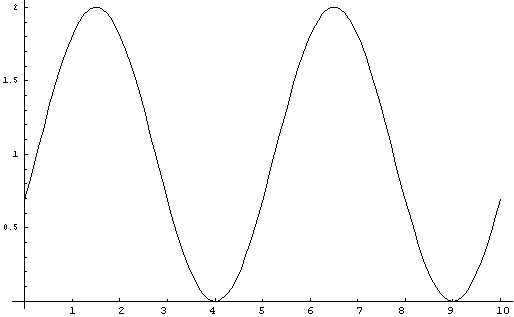Figure 14. Equation L. y = 1+Cos(Pi(2x+7)/5)

y = 1+Cos(Pi(2x+9)/5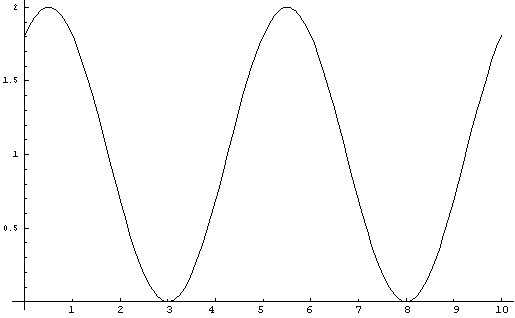Figure 15. Equation M. y = 1+Cos(Pi(2x+9)/5)

y = 1+Cos(Pi(2x+11)/5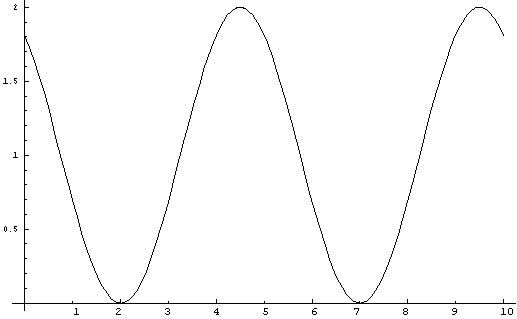Figure 16. Equation N. y = 1+Cos(Pi(2x+11)/5)

y = 1+Cos(Pi(2x+13)/5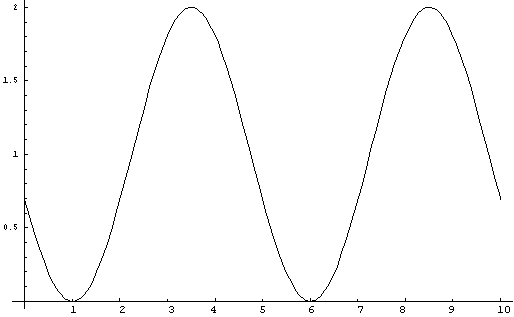Figure 17. Equation O. y = 1+Cos(Pi(2x+13)/5)

When the four equations are multiplied together the resulting equation is:

y = 1 + Cos[Pi(7 + 2x)/5] + Cos[Pi(9 + 2x)/5] + Cos[Pi(7 + 2x/5] * Cos[Pi(9 + 2x)/5] +
Cos[Pi(11 + 2x)/5] + Cos[Pi(7 + 2x)/5] * Cos[Pi(11 + 2x)/5] +
Cos[Pi(9 + 2x)/5] * Cos[Pi(11 + 2x)/5] +
Cos[Pi(7 + 2x)/5] * Cos[Pi(9 + 2x)/5] * Cos[Pi(11 + 2x)/5] +
Cos[Pi(13 + 2x)/5] + Cos[Pi(7 + 2x)/5] * Cos[Pi(13 + 2x)/5] +
Cos[Pi(9 + 2x)/5] * Cos[Pi(13 + 2x)/5] +
Cos[Pi(7 + 2x)/5] * Cos[Pi(9 + 2x)/5] * Cos[Pi(13 + 2x)/5] +
Cos[Pi(11 + 2x)/5] * Cos[Pi(13 + 2x)/5] +
Cos[Pi(7 + 2x)/5] * Cos[Pi(11 + 2x)/5] * Cos[Pi(13 + 2x)/5] +
Cos[Pi(9 + 2x)/5] * Cos[Pi(11 + 2x)/5] * Cos[Pi(13 + 2x)/5)] +
Cos[Pi(7 + 2x)/5] * Cos[Pi(9 + 2x)/5] * Cos[Pi(11 + 2x)/5] * Cos[Pi(13 + 2x)/5]
The graph for this is: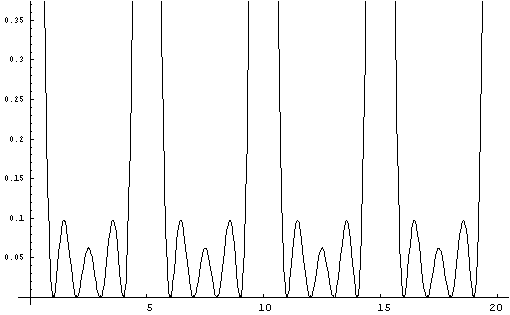Figure 18. Equation P.
y = 1 + Cos[Pi(7 + 2x)/5] + Cos[Pi(9 + 2x)/5] + Cos[Pi(7 + 2x/5] * Cos[Pi(9 + 2x)/5] +
Cos[Pi(11 + 2x)/5] + Cos[Pi(7 + 2x)/5] * Cos[Pi(11 + 2x)/5] +
Cos[Pi(9 + 2x)/5] * Cos[Pi(11 + 2x)/5] +
Cos[Pi(7 + 2x)/5] * Cos[Pi(9 + 2x)/5] * Cos[Pi(11 + 2x)/5] +
Cos[Pi(13 + 2x)/5] + Cos[Pi(7 + 2x)/5] * Cos[Pi(13 + 2x)/5] +
Cos[Pi(9 + 2x)/5] * Cos[Pi(13 + 2x)/5] +
Cos[Pi(7 + 2x)/5] * Cos[Pi(9 + 2x)/5] * Cos[Pi(13 + 2x)/5] +
Cos[Pi(11 + 2x)/5] * Cos[Pi(13 + 2x)/5] +
Cos[Pi(7 + 2x)/5] * Cos[Pi(11 + 2x)/5] * Cos[Pi(13 + 2x)/5] +
Cos[Pi(9 + 2x)/5] * Cos[Pi(11 + 2x)/5] * Cos[Pi(13 + 2x)/5)] +
Cos[Pi(7 + 2x)/5] * Cos[Pi(9 + 2x)/5] * Cos[Pi(11 + 2x)/5] * Cos[Pi(13 + 2x)/5]

When Equation P is added to Equation K, the resulting equation when solved for zero,
yields an infinite number of values of "x" which are relatively prime with respect to
2, 3 and 5. For values of "x" less than 7², "x" is prime.
This equation is:
y = 3 + Cos[Pi(2x+5)/3] + Cos[Pi(2x+7)/3] + Cos[Pi(x)] + Cos[Pi(2x+5)/3]*Cos[Pi(2x+7)/3]
+ Cos[Pi(7 + 2x)/5] + Cos[Pi(9 + 2x)/5] + Cos[Pi(7 + 2x/5] * Cos[Pi(9 + 2x)/5] +
Cos[Pi(11 + 2x)/5] + Cos[Pi(7 + 2x)/5] * Cos[Pi(11 + 2x)/5] +
Cos[Pi(9 + 2x)/5] * Cos[Pi(11 + 2x)/5] +
Cos[Pi(7 + 2x)/5] * Cos[Pi(9 + 2x)/5] * Cos[Pi(11 + 2x)/5] +
Cos[Pi(13 + 2x)/5] + Cos[Pi(7 + 2x)/5] * Cos[Pi(13 + 2x)/5] +
Cos[Pi(9 + 2x)/5] * Cos[Pi(13 + 2x)/5] +
Cos[Pi(7 + 2x)/5] * Cos[Pi(9 + 2x)/5] * Cos[Pi(13 + 2x)/5] +
Cos[Pi(11 + 2x)/5] * Cos[Pi(13 + 2x)/5] +
Cos[Pi(7 + 2x)/5] * Cos[Pi(11 + 2x)/5] * Cos[Pi(13 + 2x)/5] +
Cos[Pi(9 + 2x)/5] * Cos[Pi(11 + 2x)/5] * Cos[Pi(13 + 2x)/5)] +
Cos[Pi(7 + 2x)/5] * Cos[Pi(9 + 2x)/5] * Cos[Pi(11 + 2x)/5] * Cos[Pi(13 + 2x)/5]
The graph for this is:Figure 19. Equation Q.
y = 3 + Cos[Pi(2x+5)/3] + Cos[Pi(2x+7)/3] + Cos[Pi(x)] + Cos[Pi(2x+5)/3]*Cos[Pi(2x+7)/3]
+ Cos[Pi(7 + 2x)/5] + Cos[Pi(9 + 2x)/5] + Cos[Pi(7 + 2x/5] * Cos[Pi(9 + 2x)/5] +
Cos[Pi(11 + 2x)/5] + Cos[Pi(7 + 2x)/5] * Cos[Pi(11 + 2x)/5] +
Cos[Pi(9 + 2x)/5] * Cos[Pi(11 + 2x)/5] +
Cos[Pi(7 + 2x)/5] * Cos[Pi(9 + 2x)/5] * Cos[Pi(11 + 2x)/5] +
Cos[Pi(13 + 2x)/5] + Cos[Pi(7 + 2x)/5] * Cos[Pi(13 + 2x)/5] +
Cos[Pi(9 + 2x)/5] * Cos[Pi(13 + 2x)/5] +
Cos[Pi(7 + 2x)/5] * Cos[Pi(9 + 2x)/5] * Cos[Pi(13 + 2x)/5] +
Cos[Pi(11 + 2x)/5] * Cos[Pi(13 + 2x)/5] +
Cos[Pi(7 + 2x)/5] * Cos[Pi(11 + 2x)/5] * Cos[Pi(13 + 2x)/5] +
Cos[Pi(9 + 2x)/5] * Cos[Pi(11 + 2x)/5] * Cos[Pi(13 + 2x)/5)] +
Cos[Pi(7 + 2x)/5] * Cos[Pi(9 + 2x)/5] * Cos[Pi(11 + 2x)/5] * Cos[Pi(13 + 2x)/5]

When Equation Q is plotted from zero to ninety,
it is obvious that it has a period of thirty (2 x 3 x 5).
Similarly the next curve would have a periodicity of 210 (2 x 3 x 5 x7).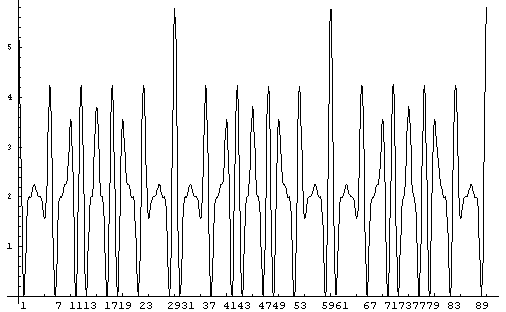Figure 20. Equation Q plotted to 90.

COMMENTARY

Are these true formulae for generating primes?
Yes.
Solve for zero and they generate integer values of all the numbers
that are prime less than a certain value.
For example: if the graphs are summed for the family of curves
up to and including 1 + Cos[Pi(A + 2x)/P(n)] then
all primes less than P(n+1) are the solutions of the equation.
Are they useful formulae?
They are computationally intensive, but the idea of multiplying
periodic equations together and solving for zero has utility value.
Suppose periodic equations could be constructed that were inherently simple
and when multiplied together were likewise simple.
The restraint of computational intensity would be diminished
with a clever enough set of periodic equations.
If non-trigonometric periodic equations could be found,
it might produce a polynomial which could be solved for
and produce integer roots all of which are composite.
From this would come all the primes via solution of a polynomial
and subsequent cataloging those integers which don't solve the equation.
Of other utility value is the possibility to demonstrate via
substitution in these equations a number's primality or compositeness
.
Again there is the computational intensiveness.
By way of example, Equation F can test
all numbers less than

10609 for primality or compositeness.
Substitute the number to be tested for 'x'.
If the answer is zero the number is composite.
If the answer is not zero the number is prime.
As mentioned before, the possibility to find simpler or
more clever periodic equations would reduce the computational intensity.
Another possibility is to choose periodic formulas where the
results can be simplified using trigonometric identities.
Whether the computational intensiveness can be reduced
enough (i.e. linear instead of multiplicative or exponential)
is an open question.